# The Hotel

The Holiday Hotel has the same number of rooms on each floor. Rooms are numbered with natural numerals sequentially from the first floor, no number is omitted, and each room has a different number. Three tourists arrived at the hotel. The first one was in room number 50 on the fourth floor. The other room number 100 on the seventh floor, third in room number 126 on the ninth floor. How many rooms are on each floor?

n =  15

### Step-by-step explanation: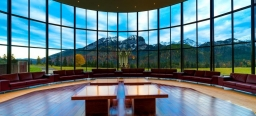Did you find an error or inaccuracy? Feel free to write us. Thank you!

Showing 1 comment:Cupcake
102–10+7Tips to related online calculators
Do you solve Diofant problems and looking for a calculator of Diofant integer equations?

## Related math problems and questions:

• There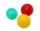There are two numbers on the screen - one in the blue and the other in the red box. In the beginning, both numbers are the same. With each beep, both numbers increase - by 1 in the blue field and by 3 in the red field. At one point, the number 49 appears
• Inverted nineIn the hotel Inverted Nine, each hotel room number is divisible by 6. How many rooms can we count with the three-digit number registered by digits 1,8,7,4,9?
• Diofant equation250x + 120y = 5640
• Exchange € 100Find out how many ways you can exchange € 100 if you have an unlimited number of 50, 20, 10 and 5 euro banknotes. Use a method other than listing all options systematically.
• One hundred stamps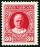A hundred letter stamps cost a hundred crowns. Its costs are four levels - twenty tenths , one crown, two-crown and five-crown. How many are each type of stamps? How many does the problem have solutions?
• Hotel 4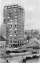A 360 room hotel has 1/3 of its rooms occupied at present. How many rooms are empty?
• Z9-I-4Kate thought a five-digit integer. She wrote the sum of this number and its half at the first line to the workbook. On the second line wrote a total of this number and its one fifth. On the third row, she wrote a sum of this number and its one nines. Fina
• The libraryAnna stored a total of 126 books in three compartments of the library. There are 6 books more in the first compartment than in the second one. The number of books in the second compartment is the arithmetic average of the number of books in the first and
• School year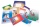At the beginning of the school year, 396 notebooks and 252 textbooks are ready to be distributed in the classroom. All pupils receive the same number of notebooks and the same amount of textbooks. How many pupils are there in the class if you know that th
• Pennies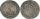800 pennies have the same value as 100 ducats. 100 pennies have the same value as 250 tolars. How many ducats has the same value as 100 tolars?
• A bookA book contains 524 pages. If it is known that a person will select any one page between the pages numbered 125 and 384, find the probability of choosing the page numbered 252 or 253.
• Pigs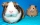A buyer said, "I want to buy 100 pigs for 100 denarii. An adult pig costs 10 denarii, a sow 5 denarii, two little piglets are worth 1 denarius. " How many pigs, sows and piglets could he buy for exactly 100 denarii?
• Four numbersThe first number is 50% second, the second number is 40% third, the third number is 20% of the fourth. The sum is 396. What are the numbers?
• Wafers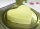They used 200 pieces to make wafers. 157 topped with caramel topping and 100 with sugar topping. How many wafers did both toppings have?
• PIN codePIN on Michael credit card is a four-digit number. Michael told this to his friend: • It is a prime number - that is, a number greater than 1, which is only divisible by number one and by itself. • The first digit is larger than the second. • The second d
• Reminder and quotientThere are given the number C = 281, D = 201. Find the highest natural number S so that the C:S, D:S are with the remainder of 1,
• RemaindersIt is given a set of numbers { 170; 244; 299; 333; 351; 391; 423; 644 }. Divide this numbers by number 66 and determine set of remainders. As result write sum of this remainders.